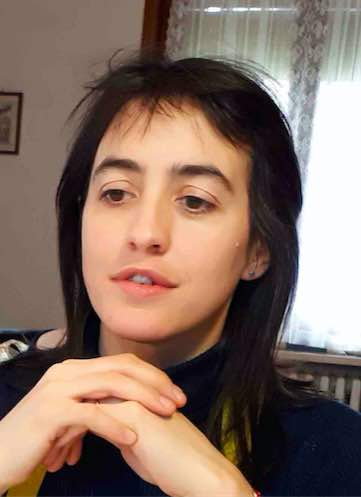# Virginia AgostinianiPosition
Temporary Assistant Professor
MAT/05 - MATHEMATICAL ANALYSIS
Research sector (ERC)
PE1_19 - Control theory and optimisation

PE1_5 - Geometry

Office
Ca' Vignal 2,  Floor 2,  Room 17
Telephone
+39 045 802 7979
E-mail
virginiaagostinianiunivritPersonal web page

#### Office Hours

Tuesday, Hours 11:00 AM - 1:00 PM,   Ca' Vignal 2, Floor 2, room 17
Alternatively, appointments can be arranged via email

Curriculum

My research activity is in the field of the Calculus of Variations and of the Analysis of PDEs. In particular, I study variational problems arising in the modelling of soft active materials. A big part of my research is also devoted to the study of geometric properties of elliptic PDEs, in the framework of the classical potential theory and in general relativity.

#### Research groups

INdAM - Unità di Ricerca dell'Università di Verona
Questa pagina è dedicata all'unità di ricerca INdAM dell'Università di Verona.
Skills
Topic Description Research area
Manifolds Geometric variational and evolution problems: minimal surfaces, motion by mean curvature. Optimal mass transport theory. Mathematics - applications and modelling
Calculus of variations and optimal control; optimization
Optimality conditions. Asymptotics of variational problems. Variational convergences and Gamma Convergence. Singular perturbations of variational problems. Mathematics - applications and modelling
Calculus of variations and optimal control; optimization
Variational problems and applications to materials science Variational problems arising in linearised and nonlinear elasticity, mathematical modelling of soft active materials Mathematics - applications and modelling
Calculus of variations and optimal control; optimization
Geometric properties of PDEs Overdetermined boundary value problems in Euclidean as well as in non-Euclidean settings, geometric inequalities, monotonicity formulas, Riemannian geometry techniques. Partial Differential Equations